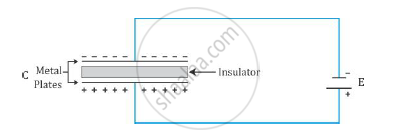# Explain Briefly the Process of Charging a Parallel Plate Capacitor When It is Connected Across a D.C. Battery - Physics

Explain briefly the process of charging a parallel plate capacitor when it is connected across a d.c. battery

#### SolutionConsider a parallel plate capacitor connected across a d.c. battery as shown in the figure. The electric current will flow through the circuit. As the charges reach the plate, the insulating gap does not allow the charges to move further; hence, positive charges get deposited on one side of the plate and negative charges get deposited on the other side of the plate. As the voltage begins to develop, the electric charge begins to resist the deposition of further charge. Thus, the current flowing through the circuit gradually becomes less and then zero till the voltage of the capacitor is exactly equal but opposite to the voltage of the battery. This is how the capacitor gets charged when it is connected across a d.c. battery.

Concept: The Parallel Plate Capacitor
Is there an error in this question or solution?